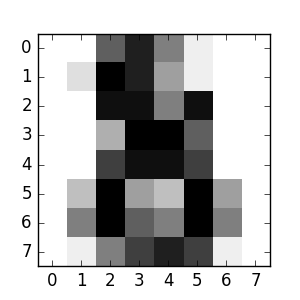# The Digit Dataset¶

This dataset is made up of 1797 8x8 images. Each image, like the one shown below, is of a hand-written digit. In order to utilize an 8x8 figure like this, we’d have to first transform it into a feature vector with length 64.```print(__doc__)

# Code source: Gaël Varoquaux
# Modified for documentation by Jaques Grobler

from sklearn import datasets

import matplotlib.pyplot as plt

Download Python source code: `plot_digits_last_image.py`
Download IPython notebook: `plot_digits_last_image.ipynb`